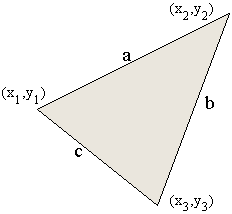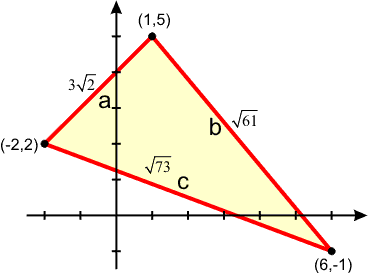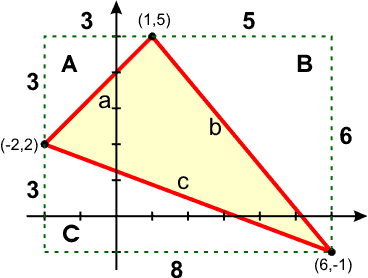# 6.5 - Applications of Matrices and Determinants

##Area of a Triangle

Consider a triangle with vertices at (x1,y1), (x2,y2), and (x3,y3). If the triangle was a right triangle, it would be pretty easy to compute the area of the triangle by finding one-half the product of the base and the height.

However, when the triangle is not a right triangle, there are a couple of other ways that the area can be found.

### Heron's FormulaIf you know the lengths of the three sides of the triangle, you can use Heron's Formula to find the area of the triangle.

In Heron's formula, s is the semi-perimeter (one-half the perimeter of the triangle).

s = 1/2 ( a + b + c )
Area = sqrt ( s ( s-a) ( s-b) ( s-c) )

Consider the triangle with vertices at (-2,2), (1,5), and (6,1).

Using the distance formulas, we can find that the lengths of the sides (arbitrarily assigning a, b, and c) are a = 3 sqrt(2), b = sqrt(61), and c = sqrt(73).

Using those values gives us ...

s = 1/2 ( 3 sqrt(2) + sqrt(61) + sqrt(73) )
s - a = 1/2 ( - 3 sqrt(2) + sqrt(61) + sqrt(73) )
s - b = 1/2 ( 3 sqrt(2) - sqrt(61) + sqrt(73) )
s - c = 1/2 ( 3 sqrt(2) + sqrt(61) - sqrt(73) )

s ( s - a ) ( s - b ) ( s - c ) = 1089 / 4

When you take the square root of that, you get 33/2, so the area of that triangle is 16.5.

#### Problems with Heron's Formula include

• Must know the lengths of the sides of the triangle. If you don't then you have to use the distance formula to find the lengths of the sides of the triangle.
• You have to compute the semi-perimeter, so chances are you will have fractions to work with.
• Lots of square roots are involved. For the lengths of the sides of the triangle and for the area of the triangle.
• It's not the easiest thing in the world to work with.

### Geometric TechniqueThe triangle can be enclosed in a rectangle. The vertices of the triangle will intersect the rectangle in three places, forming three right triangles. These triangles are denoted A, B, and C in the picture.

The area of the triangle we desire will be the area of the rectangle minus the areas of the three triangles.

The legs of the three triangles can be found by simple subtraction of coordinates and then used to find the area since the area of a triangle is one-half the base times the height.

Area of triangle A = 3 ( 3 ) / 2 = 9/2.
Area of triangle B = 5 ( 6 ) / 2 = 15.
Area of triangle C = 8 ( 3 ) / 2 = 12.

The sum of the areas of the triangles is 9/2 + 15 + 12 = 63 / 2 or 31.5.

The area of a rectangle is base times height, so the bounding rectangle has area = 8 ( 6 ) = 48.

The area of the triangle in the middle is the difference between the rectangle and the sum of the areas of the three outer triangles.

Area of triangle = 48 - 31.5 = 16.5.

Wow, that was much easier.

### Determinants

It turns out that the area of a triangle can also be found using determinants. The derivation of the formula is kind of long and most of you don't care to see it, so it's on a separate page.

What you do is form a 3×3 determinant where the first column are the x's for all the points, the second column are the y's for all the points, and the last column is all ones.

x y 1
point 1 -2 2 1
point 2 1 5 1
point 3 6 -1 1

Evaluate that determinant. I'll expand on column 1.

 -2 2 1 1 5 1 = + (-2) 5 1 - 1 2 1 + 6 2 1 6 -1 1 -1 1 -1 1 5 1

= -2 ( 5 + 1 ) - 1 ( 2 + 1 ) + 6 ( 2 - 5 ) = -2 ( 6 ) - 1 ( 3 ) + 6 ( -3 ) = -12 - 3 - 18 = -33.

It is possible that you will get a negative determinant, like we did here. Don't worry about that. The sign is determined by the order you put the points in and can be easily changed just by switching two rows of the determinant. Area, on the other hand, can't be negative, so if you get a negative, just drop the sign and make it positive. Finally, divide it by 2 to find the area.

| -33 | = 33
33 / 2 = 16.5, which was the area.

#### Formula for the area of a triangle using determinants

 x1 y1 1 Area = ± 1/2 x2 y2 1 x3 y3 1

The plus/minus in this case is meant to take whichever sign is needed so the answer is positive (non-negative). Do not say the area is both positive and negative.

Why not use absolute value, you ask? Well, think how confusing it would be to have the absolute value of a determinant.

## Test for Collinear Points

Continue with the idea of finding the area of a triangle. If the area of a triangle was equal to zero, then there would be no triangle, the points would be collinear (on a line).

We can use that fact to develop a test for collinear points.

If the area of a triangle is zero, the points are collinear.

We can drop the plus/minus and the one-half from the front of the determinant, because the area will be zero only if the determinant is zero.

Three points are collinear if and only if the determinant found by placing the x-coordinates in the first column, the y-coordinates in the second column, and one's in the third column is equal to zero.

 x1 y1 1 Does x2 y2 1 equal 0? x3 y3 1

You are not setting the determinant equal to zero, you are testing to see if the determinant is zero.

## Equation of a Line

Three points are collinear if and only if that determinant is zero.

You can force three points to be collinear by setting the determinant equal to zero.

Notice this time, that the actual variables x and y are in the determinant. That is because you have two points on a line given, and the point (x,y) is a generic point on the line.

When you expand this, I strongly recommend that you expand along the first row. That way, your multiplications to find the determinants won't involve x or y.

 x y 1 x1 y1 1 = 0 x2 y2 1

## Cramer's Rule

The derivation of Cramer's Rule can be found on another page. Here are the results.

Solve the system of linear equations

```3x + 5y = 12
2x - 4y =  9```

Let D be the determinant of the coefficient matrix.

x y
D = 3 5 = - 22
2 -4

Let Dx be the determinant of the coefficient matrix where the x column has been replaced by the constants from the right hand side.

rhs y
Dx = 12 5 = - 93
9 -4

Let Dy be the determinant of the coefficient matrix where the y column has been replaced by the constants from the right hand side.

x rhs
Dy = 3 12 = 3
2 9

As long as D, the determinant of the coefficient matrix, is not 0, then x = Dx / D and y = Dy / D.

In this case, D = -22, so Cramer's Rule will work and
x = Dx / D = -93 / -22 = 93/22
y = Dy / D = 3 / -22 = - 3/22

Cramer's rule is named after Gabriel Cramer who lived from 1704 - 1752. He is not the one who developed the technique originally, however, as the Chinese were known to have used the method before him.

### Extending Cramer's Rule to Larger Systems

Cramer's Rule can be extended to larger systems in the same manner. Simply replace each column in the coefficient matrix by the right hand side, and then divide that determinant by the determinant of the coefficient matrix.

For a 3×3 system, then let Dz be the determinant of the coefficient matrix where the z column has been replaced by the constants from the right hand side.

### Benefits of Cramer's Rule

• Determinants can be found on a calculator for large systems
• If you need just one variable, you can find it with Cramer's Rule, whereas with the other techniques you would have to find all of the variables.

### Problems with Cramer's Rule

• Takes a long time to re-enter each matrix into the calculator to find the determinant. Best suited for a computer or calculator program.
• Requires a square system.
• Doesn't always work. If the determinant of the coefficient matrix is zero, the technique fails and it is either no solution or many solutions.
• If D=0 and Dx=0 and Dy=0 then there are many solutions. You won't be able to tell what the solutions are from Cramer's Rule, but there are many solutions. This is the dependent case.
• If D=0, but at least one of the other determinants is not zero, then there is no solution. This is the inconsistent case.

## Cryptography

Cryptography involves encrypting data so that a third party can not intercept and read the data.

In the early days of satellite television, the video signals weren't encrypted and anyone with a satellite dish could watch whatever was being shown. Well, this didn't work because all of the networks using satellites didn't want the satellite dish owners to be able to receive their satellite feed for no cost while cable subscribers had to pay for the channel, they were losing money. So, they started encrypting the video signal with a system called Videocipher (later replaced by Videocipher II).

What the Videocipher encryption system did was to convert the signal into digital form, encrypt it, and send the data over the satellite. If the satellite dish owner had a Videocipher box, and paid for the channel, then the box would descramble (unencrypt) the signal and return it to its original, useful form.

This was done by using a key that was invertible. It was very important that they key be invertible, or there would be no way to return the encrypted data to its original form.

The same thing can be done using matrices.

### Encryption Process

1. Convert the text of the message into a stream of numerical values.
2. Place the data into a matrix.
3. Multiply the data by the encoding matrix.
4. Convert the matrix into a stream of numerical values that contains the encrypted message.

#### Example

Consider the message "Red Rum"

A message is converted into numeric form according to some scheme. The easiest scheme is to let space=0, A=1, B=2, ..., Y=25, and Z=26. For example, the message "Red Rum" would become 18, 5, 4, 0, 18, 21, 13.

This data was placed into matrix form. The size of the matrix depends on the size of the encryption key. Let's say that our encryption matrix (encoding matrix) is a 2x2 matrix. Since I have seven pieces of data, I would place that into a 4x2 matrix and fill the last spot with a space to make the matrix complete. Let's call the original, unencrypted data matrix A.

 18 5 A = 4 0 18 21 13 0

There is an invertible matrix which is called the encryption matrix or the encoding matrix. We'll call it matrix B. Since this matrix needs to be invertible, it must be square.

This could really be anything, it's up to the person encrypting the matrix. I'll use this matrix.

 B = 4 -2 -1 3

The unencrypted data is then multiplied by our encoding matrix. The result of this multiplication is the matrix containing the encrypted data. We'll call it matrix X.

 67 -21 X = A B = 16 -8 51 27 52 -26

The message that you would pass on to the other person is the the stream of numbers 67, -21, 16, -8, 51, 27, 52, -26.

### Decryption Process

1. Place the encrypted stream of numbers that represents an encrypted message into a matrix.
2. Multiply by the decoding matrix. The decoding matrix is the inverse of the encoding matrix.
3. Convert the matrix into a stream of numbers.
4. Conver the numbers into the text of the original message.

#### Example

The message you need to decipher is in the encrypted data stream 67, -21, 16, -8, 51, 27, 52, -26.

The encryption matrix is not transmitted. It is known by the receiving party so that they can decrypt the message. Other times, the inverse is known by the receiving party. The encryption matrix can not be sent with the data, otherwise anyone could grab the data and decode the information. Also, by not having the decoding matrix, someone intercepting the message doesn't know what size of matrix to use.

The receiving end gets the encrypted message and places it into matrix form.

 67 -21 X = 16 -8 51 27 52 -26

The receiver must calculate the inverse of the encryption matrix. This would be the decryption matrix or the decoding matrix.

 B-1 = 0.3 0.2 0.1 0.4

The receiver then multiplies the encrypted data by the inverse of the encryption matrix. The result is the original unencrypted matrix.

 18 5 A = X B-1 = 4 0 18 21 13 0

The receiver then takes the matrix and breaks it apart into values 18, 5, 4, 0, 18, 21, 13, 0 and converts each of those into a character according to the numbering scheme. 18=R, 5=E, 4=D, 0=space, 18=R, 21=U, 13=M, 0=space.

Trailing spaces will be discarded and the message is received as intended: "RED RUM"